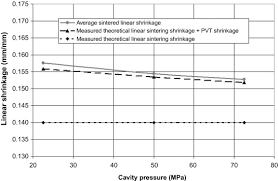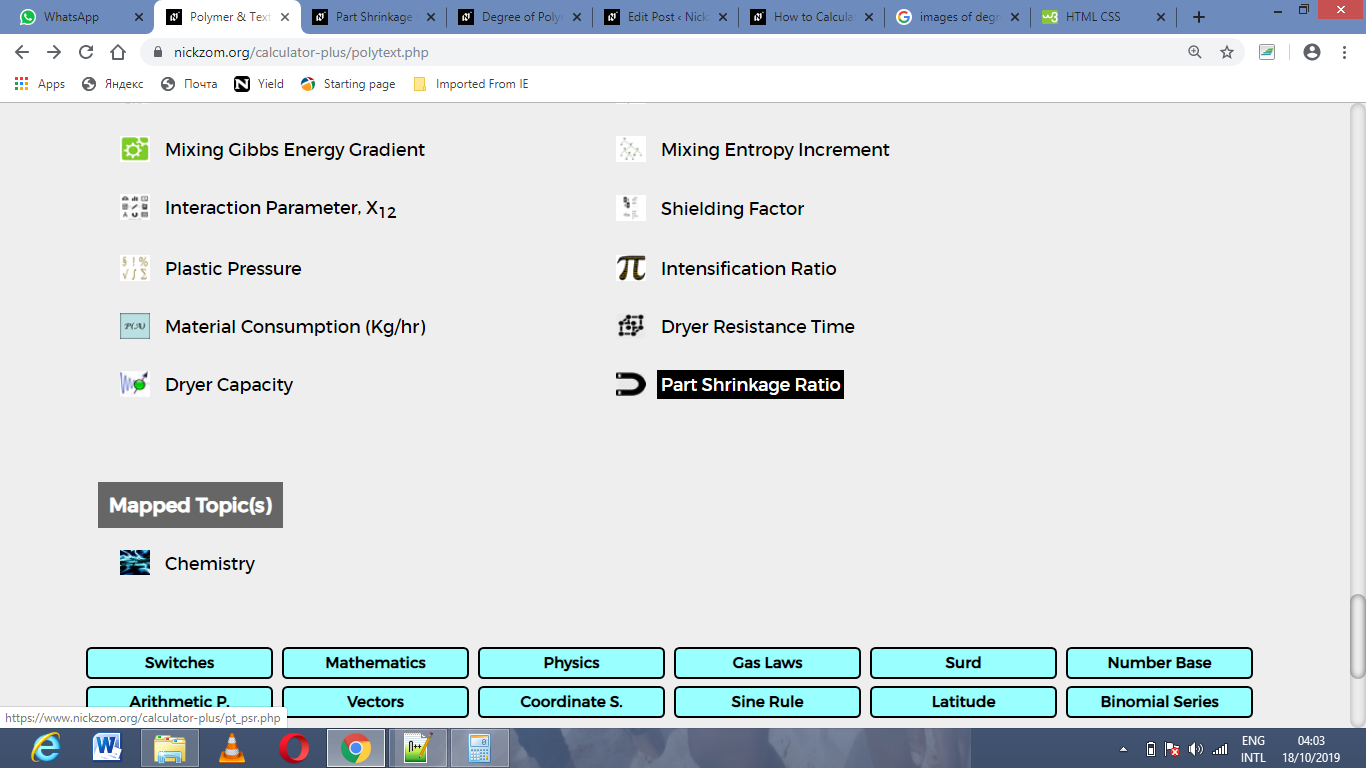# How to Calculate and Solve for Part Shrinkage Ratio | Polymer & TextileThe image above represents part shrinkage ratio.

To compute for part shrinkage ratio, two essential parameters are needed and these parameters are mold dimension (DM) and part dimension (DP).

The formula for calculating part shrinkage ratio:

RPS = DM – DP / DM

Where:

RPS = Part Shrinkage Ratio
DM = Mold Dimension
DP = Part Dimension

Let’s solve an example;
Find the part shrinkage ratio when the mold dimension is 8 and the part dimension is 10.

This implies that;

DM = Mold Dimension = 8
DP = Part Dimension = 10

RPS = DM – DP / DM
RPS = 8 – 10 / 8
RPS = -2 / 8
RPS = -0.25

Therefore, the part shrinkage ratio is -0.25.

Nickzom Calculator – The Calculator Encyclopedia is capable of calculating the part shrinkage ratio.

To get the answer and workings of the part shrinkage ratio using the Nickzom Calculator – The Calculator Encyclopedia. First, you need to obtain the app.

You can get this app via any of these means:

To get access to the professional version via web, you need to register and subscribe for NGN 1,500 per annum to have utter access to all functionalities.
You can also try the demo version via https://www.nickzom.org/calculator

Apple (Paid) – https://itunes.apple.com/us/app/nickzom-calculator/id1331162702?mt=8
Once, you have obtained the calculator encyclopedia app, proceed to the Calculator Map, then click on Polymer & Textile under Engineering.Now, Click on Part Shrinkage Ratio under Polymer & TextileThe screenshot below displays the page or activity to enter your values, to get the answer for the part shrinkage ratio according to the respective parameters which are the mold dimension (DM) and part dimension (DP).Now, enter the values appropriately and accordingly for the parameters as required by the mold dimension (DM) is 8 and part dimension (DP) is 10.Finally, Click on CalculateAs you can see from the screenshot above, Nickzom Calculator– The Calculator Encyclopedia solves for the part shrinkage ratio and presents the formula, workings and steps too.